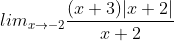# Basic maths-calculus-Q2

+1 voteThe value is

(A) 1

(B) -1

(C) 0

(D) does not existasked May 19
reshown May 24

Right limit :Since we are finding right limit, hence,    ;  ; Since,h is positive,so, |h| = h

= 1

Left limit :Since, h is positive, so, |-h| = h

= -1

So, left limit is Not same as right limit, hence, limit does not exist.answered May 27 by (112,760 points)answered May 26 by (7,690 points)answered May 27 by (180 points)